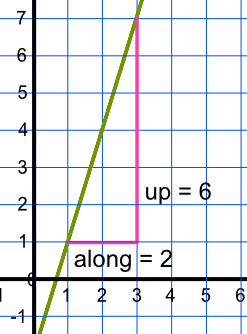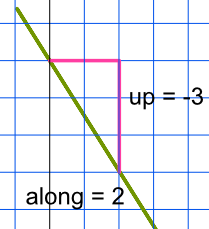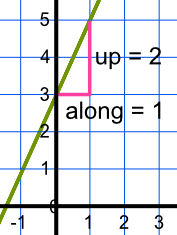y=mx+c

# y=mx+c

GCSE(F), GCSE(H),

The gradient, or slope, of a straight line is given by frac(text(up))(text(along)). In the example below, the line moves 6 up for each 2 along, and the gradient is frac(6)(2) = 3.A gradient can be positive or negative. A line falling from left to right gives a negative gradient:For each 2 along, the line falls by 3. This gives a gradient of frac(-3)(2) = -1.5. If a gradient is zero, it is horizontal. If a gradient is divided by 0, then it is vertical.

A straight line can be written as y = mx + c: m is the gradient, c is the intercept on the y-axis:The gradient of the line is frac(2)(1) = 2, which is the m in y = mx + c. The c is where the line crosses x = 0: in this example the line crosses x = 0 with a value of y = 3. The equation is y = 2x + 3.

## Examples

1. What is the gradient and intercept of the line y = -4x - 3?

Answer: The gradient is -4; the intercept is -3

Using y = mx + c, m = -4 and c = -3.

2. Two points on a line are (0, 4) and (1, 7). What is the equation of the line?

Answer: y = 3x + 4

The gradient is frac(3)(1): the line moves up 3 for each 1 along. This gives the m value as y = 3x + c.

For the intercept: when x = 0, y = 4, 4 = 3 x 0 + c therefore c = 4.